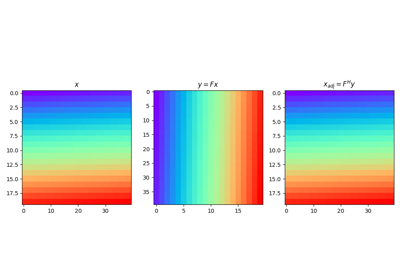# pylops.Transpose#

class pylops.Transpose(dims, axes, dtype='float64', name='T')[source]#

Transpose operator.

Transpose axes of a multi-dimensional array. This operator works with flattened input model (or data), which are however multi-dimensional in nature and will be reshaped and treated as such in both forward and adjoint modes.

Parameters
dimstuple, optional

Number of samples for each dimension

axestuple, optional

Direction along which transposition is applied

dtypestr, optional

Type of elements in input array

namestr, optional

New in version 2.0.0.

Name of operator (to be used by pylops.utils.describe.describe)

Raises
ValueError

If axes contains repeated dimensions (or a dimension is missing)

Notes

The Transpose operator reshapes the input model into a multi-dimensional array of size dims and transposes (or swaps) its axes as defined in axes.

Similarly, in adjoint mode the data is reshaped into a multi-dimensional array whose size is a permuted version of dims defined by axes. The array is then rearragned into the original model dimensions dims.

Attributes
shapetuple

Operator shape

explicitbool

Operator contains a matrix that can be solved explicitly (True) or not (False)

Methods

 __init__(dims, axes[, dtype, name]) adjoint() apply_columns(cols) Apply subset of columns of operator cond([uselobpcg]) Condition number of linear operator. conj() Complex conjugate operator div(y[, niter, densesolver]) Solve the linear problem $$\mathbf{y}=\mathbf{A}\mathbf{x}$$. dot(x) Matrix-matrix or matrix-vector multiplication. eigs([neigs, symmetric, niter, uselobpcg]) Most significant eigenvalues of linear operator. matmat(X) Matrix-matrix multiplication. matvec(x) Matrix-vector multiplication. reset_count() Reset counters rmatmat(X) Matrix-matrix multiplication. rmatvec(x) Adjoint matrix-vector multiplication. todense([backend]) Return dense matrix. toimag([forw, adj]) Imag operator toreal([forw, adj]) Real operator tosparse() Return sparse matrix. trace([neval, method, backend]) Trace of linear operator. transpose()

## Examples using pylops.Transpose#Transpose

Transpose